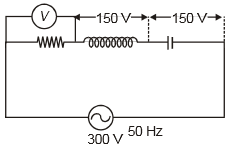In the circuit given below, the reading of the voltmeter V will be1.  0

2.  100 V

3.  200 V

4.  300 V

Concept Questions :-

Different types of AC Circuits
High Yielding Test Series + Question Bank - NEET 2020

Difficulty Level:

If the current in an AC circuit is given as $\mathrm{i}=\left(5\mathrm{A}\right)\mathrm{sin}\left(\mathrm{\omega t}-\frac{\mathrm{\pi }}{3}\right)$ when an emf $\mathrm{e}=\left(200\mathrm{V}\right)\mathrm{sin\omega t}$ is applied across the circuit. The circuit has components-

1.  L and C

2.  L and R

3.  C and R

4.  None of these

Concept Questions :-

Different types of AC Circuits
High Yielding Test Series + Question Bank - NEET 2020

Difficulty Level:

A step-down transformer is employed to reduce the main supply of AC from 220V to 11V. If the primary coil draws a current of 5A and the secondary supplies a current of 90A, then the efficiency of the transformer is  -

1.  10%

2.  95%

3.  90%

4.  80%

Concept Questions :-

Transformer
High Yielding Test Series + Question Bank - NEET 2020

Difficulty Level:

At resonance, the value of the power factor of the circuit is -

1.  1

2.  Zero

3.  Between zero and 1

4.  More than 1

Concept Questions :-

LC oscillations
High Yielding Test Series + Question Bank - NEET 2020

Difficulty Level:

In a LCR circuit having L = 8.0 henry, C = 0.5 $\mathrm{\mu F}$ and R = 100 $\mathrm{\Omega }$ in series, the resonance frequency (in Hz) is

1.

2.

3.

4.

Concept Questions :-

LC oscillations
High Yielding Test Series + Question Bank - NEET 2020

Difficulty Level:

In a LCR series network, . The supply voltage is

1.  25V

2.  75V

3.  35V

4.  Zero

Concept Questions :-

Different types of AC Circuits
High Yielding Test Series + Question Bank - NEET 2020

Difficulty Level:

The ratio of the number of turns in secondary to primary in an ideal transformer varies from 50 to 550. If the power input is P, then its output power is  -

1.  11P

2.  $\frac{\mathrm{P}}{11}$

3.  $\frac{18P}{111}$

4.  P

Concept Questions :-

Transformer
High Yielding Test Series + Question Bank - NEET 2020

Difficulty Level:

Loss in energy in the transformer is due to

1.  Hysteresis

2.  Eddy current loss

3.  Leakage of flux

4.  All of these

Concept Questions :-

Transformer
High Yielding Test Series + Question Bank - NEET 2020

Difficulty Level:

1.

2.

3.

4.

Concept Questions :-

Rms and average values
High Yielding Test Series + Question Bank - NEET 2020

Difficulty Level:

In a step-up transformer, the turn ratio is 1:2. A Leclanche cell (e.m.f. 1.5V) is connected across the primary coil. The voltage developed in the secondary coil would be-

1. 3.0 V

2. 0.75 V

3. 1.5 V

4. Zero

Concept Questions :-

Transformer